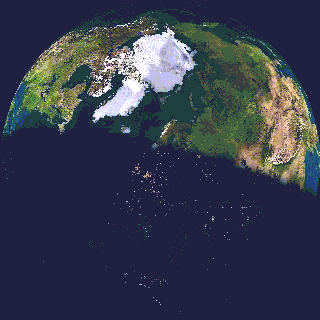ON THE NATURE OF

GRAVITATIONAL ATTRACTION

## INTRODUCTION   (an article)

1. According to experimental data and the laws of motion, it is imperative that the gravitational attraction shall be greater at the equator then anywhere else upon the earth, since conclusively the force with which a person or an object is driven away from the center of the earth (centrifugal force) is greatest at the equator.

2. Since then the weight of an object remains the same anywhere upon the earth, gravitational force is greatly susceptible and augmented to by the centrifugal force.  Accordingly, a natural as well as a logical concept in the nature of gravitational force is needed and herewith implemented.

3. This new concept brings the natural forces and movements of all physical nature into harmony with one another, whereby gravitational force (as an inclination to downward movement), becomes unique as the net result of a sequence of events.

Concepts of gravity.

1. When Sir Isaac Newton realized that there must be a force to pull or drive down the apple from the tree, he weighed the thought that there were mechanics in every move.

2. Such thoughts, although not new, seem nonetheless rare.  Deep thinking, as we call it, to realize upon fundamentals - that it is not of itself that we walk upon the earth, or even to walk upright, is not common place.

3. Thought therefore, to conceive fundamental thought, is as one might say a profound measure of intelligence, and curiosity a quadroon of that measure.  But not all thought however comes to make a home run.

4. Newton's concept for the unseen and unknown force of gravity seated itself in the mass of the objects:  "Every mass having a force where-with it attracts every other mass". (Figure 44-1).

5. Sir Isaac Newton should have known that his law of gravitation did not jibe with his other law of gravitation wherein an object spins outward towards the centrifugal.

6. This is so since he mentions mass or weight, - and there can be no measure of weight when two forces pull equally to opposite directions.  And how is weight found to exist?

7. Einstein on the other hand had to reconcile himself with the null result of an "ether" experiment to establish a medium for light to travel upon.  The same ultimately led him to contrive the theory of relativity in which gravity became the essence of an object falling into the depression of warped space (Figure 44-2).

8. My intend however is not to debate the validity of either of these concepts, but to take a closer look at our own textbook law of gravity (Figure 44-3), and from that law bring the nature of gravitational force to bear.

A law of inward moving force.

1. Newton at a young age formulated a law of gravitation:  "Everybody in the universe attracts every other body with a force proportional to their masses, and inversely proportional to the distance separating them."

2. And with it the means to calculate the force:  "The gravitational acceleration of a mass in  uniform circular motion is proportional to the square of its speed and inversely to the radius of its path".

3. Now we must stop since these two law contradict one another. This is so since the second law is based on inertia, and the inertia of any mass depends upon its magnetic hold as well as its velocity. Therefore the contradiction.

4. Then again, according to Newton:  "The centripetal acceleration of the moon and the downward acceleration of "g"' of the objects dropped at the earth's surface. have an identical cause, namely - gravitational attraction."

5.   Consequently, it is without question that the gravitational force wherewith every part is attracted to the surface of the earth varies from equator to pole, since again without question, the centrifugal force has a greater impact upon an object at the equator than at those near any polar region.

6. It is to be noted here that the term "acceleration" does not fit in the above statements, the proper term being "movement" or constant thereof.

7. If - when traveling from Alaska to Equador - we gradually gained weight, that would be conclusive evidence that gravitational force is "not" susceptible to the centrifugal force.

8. Since however our weight remains the "same" no matter where we may place ourselves upon the earth, this in itself confirms that centrifugal force directly affects gravitational pull, and that it compensates for its net outcome by augmenting it.

9. Gravitational force and centrifugal force thus are relative to one another.  And to state a "law" of gravity, it should read as follows:

10. "Gravitational force is directly proportional and augmented to by the centrifugal force (inertia) of any object in uniform circular motion."

11. The same law then applies equally to anything "centripetal" to value according to the square of its speed and inversely so to the radius of its path.  The "new" law of gravitation must thus include the "augmentation" of the "inertia" of the bodies.  This one thing Newton failed to include.  The law may thus be stated as:

12. Every body in the universe attracts every other body with a force directly proportional to the sum square of their inertia, and inversely proportional to the square of the distance separating them.

Gravity verses centrifugal

1. Reference  figure 44-4:   The intimate relation of gravitational attraction with centrifugal force should be as clear as two plus two to equal to four.  Susan at Ecuador has a net weight of 110 lb.  Her inertia in terms of centrifugal force (F) will drive her away from the earth by an exam plenary figure of 100 lb.  The gross gravitational force upon her must thus be 210 lb.

2. Her vacation now being ended, she returns to Alaska where her inertia (F) decreases to one half since her radius is reduced by one half, and consequently the square of her speed is reduced likewise.

3. The sum will then be 50 lb. of inertia as compared to 100 previously.  Susan still reading her weight at 110 lb. has now a gross gravitational attraction upon her of only 160-lb.

4. Centrifugal impact has a direct effect on the gravitational force wherewith the mass is drawn to the center of the earth.  In no other way could Susan have read her weight the same at both places.  Gravitational force therefore, to put it another way, is directly susceptible to the "inertia" of all bodies rather than the "mass" thereof.

5. As a general rule, an increase in mass is also an increase in inertia.  This however as a general rule is deceptive, since inertia is not the same as mass.  A one (1) lb. object in rest position does not have the same inertial value as when it is traveling along a linear or uniform circular path, the speed of which squares the force, or inertia - as the same may be said.

6. Up to this point we have established "two" factors relative to gravitational attraction, "centrifugal force", and "inertia" both augmenting gravitational pull.  But now comes the question how inertia together with its centrifugal cousin becomes a centripetal force the nature of gravity.

Inertia into precession.

1. We can view an object of mass as being a "gyro", or as consisting of gyro's since all fundamental parts (atoms) are in fact gyro's.  The gyro then seems to have the ability to defy gravitational force.  This defiance, in the nature thereof, is in fact the reason why all objects come under the spell of an inward moving force.

2. By illustration figure 44-5,  an object with angular momentum (gyro) in a state of rest will remain in place as were it fixed to the space in which it resides.  But when moved, even in the smallest degree from the equilibrium in which it is fixed, a "torque" is placed upon that angular inertia causing it to precess, (Direction R at right angle).

3. The same is true for linear movement to place a torque upon the axis of rotation (Q to M, H) and thus again precess in the same manner.

4. This establishes that - any substance either at rest or in linear motion, when a torque is placed upon it - will precess due to its fundamental angular momentum.

5. What this all means is that our object in figure 44-5, when moved to the left will happily precess as it moves into the direction of inertia.

6. We however wish it to proceed by a uniform circular motion to follow an orbital track so that we can say; "it is under the effect of gravity."  But so far we have not as yet found sufficient reason why the object should change from a straight-line trajectory into the direction of inertia to an orbital trajectory.

7.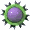So let us look at this once again, for there is still that centrifugal force and direction.  We do have a mass at angular momentum in which we placed a line to display its axis of rotation, a convenient reference by which to determine its position.

8. Let us then place our finger carefully upon this gyro and push it to our left; in other words, we have given it linear movement without disturbing the position of its axis of rotation.

9. Question now:    Can we speak of a centrifugal force existing upon it, and/or of any centripetal force existing upon it?  The answer is, no!

10. For in order to implement a centrifugal force we must fasten a string to our gyro connected to a stationary point (like point C for example at earth's center) so that it may be drawn away from its straight line movement into a circular movement.  For it is then that we come to feel the centrifugal impact it has upon us.

11. And neither shall it have centripetal or gravitational inclinations, for when there is no centrifugal, there is neither a centripetal.  And even with our string connected, and our gyro placed in a circular movement, and the definite conviction that we do have a centrifugal force pulling against us, there is still no gravitational nor centripetal force.

12. We may cause the object to come downwards to us, as in a gravitational decline by pulling on the string, but that is not a force of gravity, it is our physical force pulling it down.

13. We have not as yet seen gravity, but we noted one important established factor, which is "precession".  For when we tied our string to the gyro and caused it to go by a circular movement, we also obtained a centrifugal pull, which in turn automatically and simultaneously, induced a "torque" upon our angular inertial friend, who in turn started to "precess".

14. This precession then is "not" gravity, it is not a force moving into a downward linear direction, but in a circular formation after the angular inertia of its being.  This precession can however be turned into a factor that ultimately leads to gravity - if only we can find a helping hand, a force with the power to move things.

15. In other words, we require arms to lay a hold upon the object and its movement of precession.  And there is just such a force, a very fundamental force, very uniquely suited to just that purpose, namely,

Magnetic force

1. As we said, we need a set of strings that are susceptible to the movement of the wheels of nature, a set of strings that conduct themselves along all those many gyro's of nature.  And it must be a set of strings that have the natural ability to move things when at any time they are able to lock on to the structure of any mass by means of local motions entwined therein.

2. I believe we have just described magnetic force as the ideal entity to serve our purpose in at last establishing gravity.  Magnetic force has a multitude of strings going through all substance and embracing all substance.  And given a local formation (components) it is well suited to be intertwined with the structural grids of any mass.

3. It has the power to draw any substance at any distance to the far reaches of its tentacles.  Magnetic force therefore is the one force needed, and it is the "only" force available for us to call upon.  For again, there is no other force in nature that not in one way or another is by it, or derived from it.

4. With the answer to our needed strings, the object is then of course also directly related to the earth and to the center thereof.  At any time therefore when the object makes any movement to depart, this movement is directly felt and reacted upon by the magnetic tentacles, which as we mentioned earlier, results into a precession of the multitude of gyro's of which all substance is made-up.  This may be seen by the broken lines (magnetic lines) upon points H and M.  (figure 44.5)

5. The movement of the object to the left thus causes distance HC to increase in respect to distance MC, consequently establishing the torque.  At this stage of the game we still do not yet have a movement of gravity, the only action to which the magnetic lines of force are able at this point is to produce "somewhat" of a "strain".

6. This strain however is sufficient to induce the torque, even if it be a minimal of torque.  For as soon as a mere minimal is set-up, a centrifugal impact is also set-up which in turn increases the torque, and as the torque is increased so in turn the power to precession increases.

7. The final stage of all this scenario then is in this movement of precession firmly embraced within the magnetic lines of force turning into them.  This then is the relative movement we call "the inclination to downward movement", or in man's words, "gravity".

A motion of 7000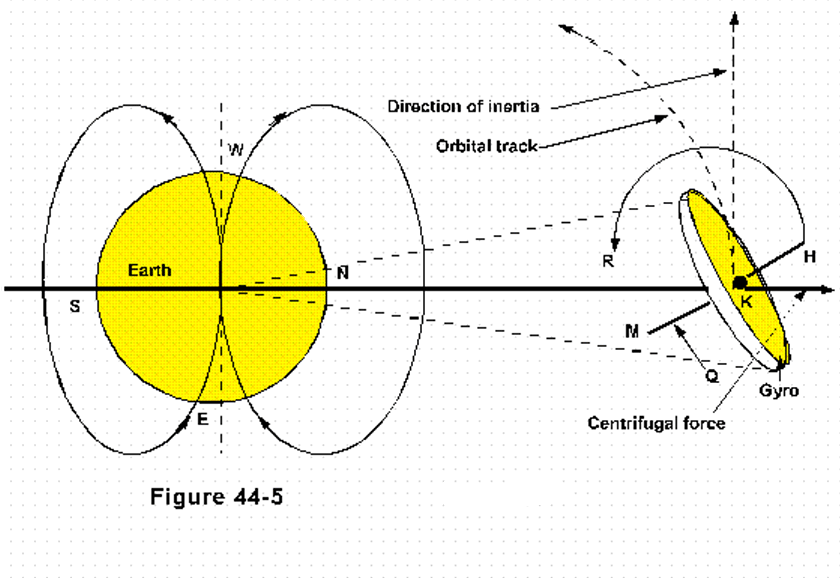1. A movement of 7000, is the essence of an angular movement after the coordinate of a figure eight, the principle of the fundamental force of nature.  I am speaking of what we should call a "component system".

2. Linear magnetic force in establishing itself within a compound such as steel utilizes a component, a local formation after its own coordinate whereby it may lock itself unto the structure of the compound.

3. And accordingly it motivates any compound with the same or similar structure.  (Steel to magnetized steel, magnet to magnet).

4. By this component factor magnetic force is thus quite able to draw such particular substances unto itself, but the same components are not suited for many other substances.

5. And so in addition to this "linear" component, there is also an "angular" component that may be drawn in any substance since it lays hold on the precessional movement of all objects, or parts having angular inertia.

6. When we speak of motion, there are two directions, angular and linear.  And when we speak of magnetism, there is angular magnetic force as electricity, and linear magnetic force as what we usually call magnetic force.  And so, as there are always two directions of the same fundamental footing, so there are two component factors, linear and angular.

7. To illustrate the inner workings of a component, linear or angular, now is easier said than done.  I do not have that precise information, but I do know the principle by which it operates.

8. And to illustrate this, the atom (or object) in figure 44-6-A, may in all respects remain stationary, the angular moment of its electrons providing the basis for a greater (component) movement that (turns) precesses within the tentacles of the "overall" force of the earth.

9.The downward movement of the substance may thus be likened to a nut turned upon a bolt.  The strings of magnetic force being the bolts, the component movement being the nut.  Or in reverse, viewing the bolts to turn, while the nut remains stationary.  Both aspects may be viewed correctly.

10. Part B, figure 44-6, is a more comprehensive illustration of the component factor, drawing a figure eight in the angular mode by which it is captured upon in the precession of nature's angular inertia.

11. At this point it should become obvious just why gravity does not draw us to the earth at the speed of light.  For if we were drawn by a gravity which does not utilize this particular component system, we would fall at the speed of light.

12. We however have the soft hands of a female force whereby we are drawn to the earth.

13. Or to rephrase it, we are taken into a immobile turn by the hands of a lady of force who delivers us into the power of her man to draw us down to his center of being.

14. For like a nut turned unto a bolt the downward velocity is slowed according to the number of turns per given length.

15. Accordingly, our maximum velocity in a gravitational descend is fixed according to that value, and our acceleration is regulated by the same.

### Earth’s center how and why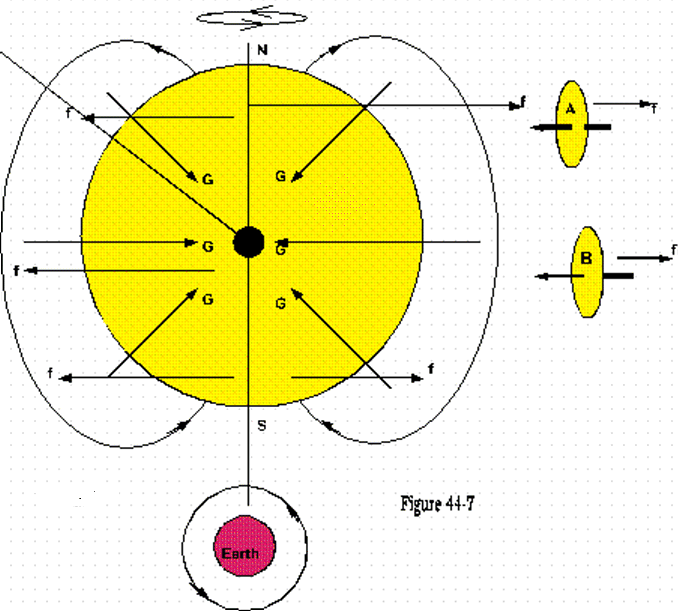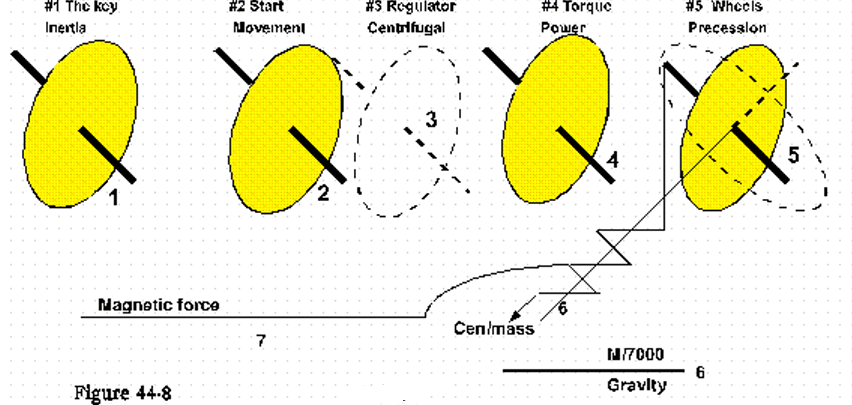1. Now that we have an inclination to downward movement and a force to pull us down, let us investigate just how and why the trajectory of that movement is to the very center of center of the earth.

2. A centrifugal; force has its vector all along the axis of rotation, wherefore the whole 8,000 mile long axis of the earth is the vector of the centrifugal force. parts A and B pointing to the axis).

3. And while basically we are not drawn to the earth by centrifugal force, we are nonetheless driven away from our home planet by that force which must be offset and overcome by our gravitational force.

4. There is then the magnetic force in its ideal figure eight which vector is not along a line, but to a center, by which all substance under the influence of a gravitational descend may be drawn.  Accordingly, by figure 44-7, the trajectory of gravity (G) is to the single center, even as the trajectory of magnetic motion is to that same single center.

5.   Gravity thus begins and ends with magnetic force.  It begins with its lines to react upon a movement of the inertia, and ends in bringing it down by a set of motions that form and act upon the precession of that inertia in the degree of torque placed upon it.

6. The center of magnetic force is situated by what is called equilibrium, movements seeking their balance in the given mass.

7. If therefore our earth were broken in two halves, there would be two separate magnetic entities each with its own newly relocated center, and a newly oriented force of gravity.

A chain of consequences.

1. There are seven factors that play a role in the making of gravity.  And we are going to make it simple by a list of sequence of events.

2. First we must note that - mass without motion - is not mass, since matter cannot be accounted for without the motion by which it is found.  The life of matter is motion, and the existence of motion is upon matter.

3. By illustration, angular momentum as "inertia" comes first, and it is the "key" factor (Figure 44-8- #-1).    Let us then assume this inertia to be in a state of rest, fixed in the space in which it resides, which in all reality is not to be found, all things in the universe being in motion.

4. Thus, now that we have "the key" (factor 1) we must "start" the vehicle, which is to bring "movement" to it (Figure 44-8- #2).

5. Movement then can be just about anything, the orbital movement of the earth around the sun, the tracking of a radius with the world as it spins the movement of the solar system as a whole, galactic movement.  And any dynamic movement such as we make in contrast to the above and relevant to the vector of gravity.

6. Any movement now will be resisted by the angular inertia, and any movement that tends to bring it from its perfect balance such as an impact of centrifugal, will effect its position.  Factor number three therefore is the "centrifugal" force, and called; the "regulator". (Figure 44-8-#3).

7. Centrifugal force then as the result of any movement that brings the inertia from its inertial balance, instantly translates into a "torque" upon the whole of the inertia, which we find convenient to demonstrate by an axis thereof.

8. The torque in all respect is "power"; it is the weight or emphasis of power bearing down upon the shafts and wheels of the vehicle.  This we come to list as factor number 4 in the sequence of events that follow in consequence of one another.

9. Factor number 5 may be called the "wheels" of the vehicle, which is the movement of precession brought about by torque upon angular momentum. (Figure 44-8- #5).

10.The next step is the embrace of magnetic force, which itself is noted as factor number 7, upon the precession in a greater, or local formation of movement which we came to call "the inclination of gravitational descend," or "M/7000," or just plain "gravity."

11. And we list it as number six, which destiny is at or towards the center of force, which most always is also the center of mass.

12. Yet another example in order to make gravity better understood.    By illustration figure 44-9, one needs a "key" to "start" the car, and place it in gear, to "regulate" it, which results into a "torque" upon the drive-shaft implementing angular momentum (precession), to the "wheels".

13. These in turn resting upon the "pavement" as "magnetic embrace" bring "linear movement" as "gravity" to the vehicle to bring it "home" as the "center" of magnetic force.

14.And to give still another example in which to emphasize the "regulator", how by the increase and decrease of centrifugal force gravity is likewise increased and/or decreased.  Factor number 1. (Figure 44-10),   is at the center of the picture, since it is the "key".

15. This key and its material structure in which it operates may be brought down into a movement called gravity by placing a torque upon its axis resulting into a complex set of spiral movements along a linear flow of magnetic force.

16. The power towards downward movement, as "the force of gravity", is then regulated by a scale called "centrifugal impact", which regulates the plus or minus movement of all such motions that may be brought upon the key.

Why a gyro defies gravity

1. Why does a stone thrown to some distance stay in the air to follow by a curve before it falls to the ground?  Answer: Linear inertia overcoming gravitational pull.  As long as the linear inertia (determined by speed) either exceeds or is equal to the force of gravity, the stone will remain in level or better than level flight.

2. The instant its inertia comes below that value it will be drawn down.  The same is true for angular inertia.  If a wheel is spun at a momentum exceeding the inertial momentum of the gravitational attraction on its parts, it will remain fixed in place, as were it in defiance of gravity.

Miscellaneous

1. True or false?  If we stopped the earth from spinning we would all float into space.

2. False, for there is yet the movement of our solar system, as well as our own orbital movement.

3. So then if we removed the inertia from every atom, would we then be free of gravity?  Yes indeed we would, but then neither our bodies nor any other physical thing would be.

4. If we removed magnetic force, would gravity cease to be?  Yes it would, we would all be riding a large comet into the far reaches of space.

5. If we applied sufficient inertia to a mass to overcome downward force (gravity) would we be weightless?  Yes and no, but the same is irrelevant, since the very thing we added adds that much more to free ourselves, unless it is linear inertia.

6. Can we somehow eliminate or neutralize centrifugal force?  No, not unless we remove inertia, which in turn removes physical being.

7. These are not fanciful statements.  Nor have I as some may presume, propounded a concept like a theory.  On the contrary these factors are the definition by which gravity is upon us.

8. It are the laws of motion asserted to by facts and evidence of experiment that cannot be bend to allow a turn into a direction that violates their very incepts.

9. And where the signposts are changed, he who travels accordingly finds no destiny.  And what is not in man's destiny, he shall not obtain.

Conclusion

1. A unified field concept becomes reality when we are served with the fundamentals of nature that home in on its natural forthcoming.  Gravitational force is a branch of that tree which like any other branch of a tree cannot survive nor exist on its own.

2. Nor need we look very far to find evidence for our concept of gravity.  The fact that centrifugal force augments gravitational pull is as conclusive as the sun to rise each morning.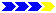Next page